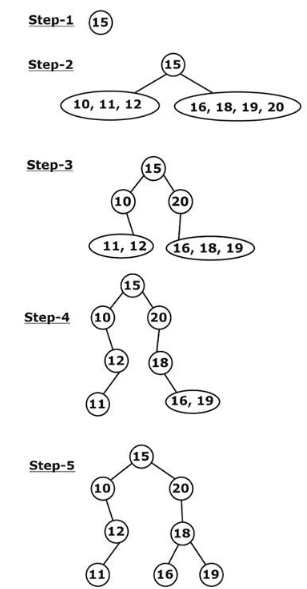# GATE Solved Paper 2020 - GATE 2020

• A

III only• B

I and IV only• C

I and III only• D

I and II only• Option : C
• Explanation :
Statement 1: True, Daisy chaining assign non-uniform priorities in attending interrupts.
Statement 2: False, A vectored interrupt means, CPU knows the source of the interrupt.
Statement 3: True, polling technique makes CPU to periodically verity states bits and service for need
Statement 4: False: During DMA also, CPU will have master control over the bus. (OR) IOP (I/O processor) and CPU can be mastered but not at the same time.
Hence, I and III are true

• A

11, 12, 10, 16, 19, 18, 20, 15• B

20, 19, 18, 16, 15, 12, 11, 10• C

10, 11, 12, 15, 16, 18, 19, 20• D

19, 16, 18, 20, 11, 12, 10, 15• Option : A
• Explanation :Post order: 11, 12, 10, 16, 19, 18, 20, 15

• A

Θ(n2)• B

Θ(1)• C

Θ(n log n)• D

Θ(n)• Option : C
• Explanation :
Inserting an element at the beginning of the linked list takes O(1) time. But, If it needs to be sorted, then in the worst case, it takes (log n) time using merge sort. To insert such n elements and make sure it is in sorted order, the worst case time complexity would be O(n log n).

• A

II and III only• B

I and III only• C

II only• D

III only• Option : D
• Explanation :
(i) e–x is decreasing

(ii) f(x) = x2 – sinx
f′ (x) = 2x – cos x
Now check f′ (x) at [0, 1]
f′ (0) = –1
f′ (1) = 2 – cos 1 = 1.4596
∴ f(x) is not increasing everywheref′(x) ≥ 0 for all x.
∴ f(x) is an increasing function.

• A

10*(0*10*10*)*• B

(0*10*10*)*10*• C

(0*10*10*)*0*1• D

((0 + 1)*1(0 + 1)*1)*10*• Option : B
• Explanation :
Eliminate the options.
--Option (A) is incorrect, it forces the string to start with 1, we cannot generate strings like 01, 0111, ….
-- Option (C) is incorrect, it generates string ending with 1, so cannot generate strings like 10, 1110
-- Option (D), not always generates strings with odd 1’s as it can generate 110.
-- Options (B), is correct, as it can generate all the string with odd number of 1’s.
Related Quiz.
GATE 2020